# Newton's Corpuscular Model of Light & Huygens' Wave Model of Light

This is part of the HSC Physics course under the topic Wave Model of Light.

### HSC Physics Syllabus

• analyse the experimental evidence that supported the models of light that were proposed by Newton and Huygens (ACSPH050, ACSPH118, ACSPH123)

### Newton’s Corpuscular Model of Light

At an early age, Newton has always been interested in the theory of light.

Newton's model of light proposes that propagation of light is caused by the rectilinear motion of light particles which he termed light corpuscles. These light particles travel at a finite speed and their interactions with the external environment e.g. rigid surfaces, walls and human eye, obey Newtonian physics.Newton thought light cannot be a wave primarily because it did not show diffraction characteristics like sound. He argued that if light is a wave, we would be able to see light when a physical barrier is placed in front of the source, analogous to how sound travels around a physical barrier to reach our ears.

He argued that light does not exhibit diffraction

‘We can hear sound from behind an obstacle, but we don’t see light’

Besides the ‘absence’ of diffraction, Newton used light’s ability to travel in a vacuum to support his particle model. At the time, all known waves required a medium to propagate (these are now defined as mechanical waves)

Additionally, he explained different colours of light by proposing each colour is encoded by a light corpuscle of a particular size.

#### Advantages of Newton’s Particle Model

• This simple theory of light propagation and composition explains the basics of optics including:
• Optics within lenses and through pinholes

Light corpuscles’ velocity remains constant unless acted upon by the external environment. Light rays change in direction within concave and convex lenses due to the nature of their surfaces. This results in the magnified or diminished and inverted or upright images.The inverted, same sized image formed when light passes through a tiny pinhole can be understood by the constant direction of the light corpuscles that travel through the hole.

• Reflection of light

Newton demonstrated that when spherical objects e.g. ball undergoes elastic collision with a rigid surface, the angle at which it collides with the surface equals to the angle at which it leaves the surface. He used this application of Newtonian physics to explain the reflection of light corpuscles when in contact with a surface.• Refraction of light

This is Newton’s least valid proposal in his corpuscular theory of light. Newton proposed that particles of a medium produce attractive forces. So, denser media have more particles and thus stronger attraction. When light corpuscles approach an interface, the stronger attraction of the denser medium produces on the corpuscles a net force and thus acceleration towards it.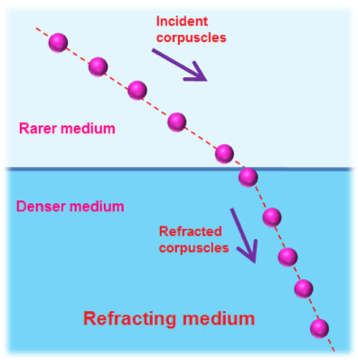This acceleration may be along (speeding up) or against (slowing down) the velocity component normal to the interface depending on whether the refracting medium is the denser one or not. And, the velocity component parallel to the interface is constant. This causes the difference in final and initial net velocities to produce the effect of light bending away or towards the normal.

#### Limitations of Newton’s Particle Model

However, Newton’s theory could not explain:

• Partial refraction and partial reflection

In his theory, he simply postulated that light corpuscles have a ‘fit’ which determines whether a particular corpuscle enters the medium (for refraction) or is reflected.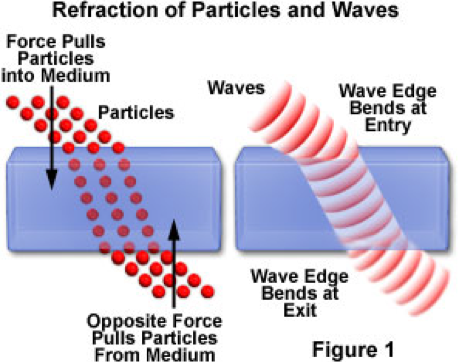Without experimental evidence, Newton acknowledged that his theory is insufficient in explaining the partial reflection and partial refraction phenomenon of light.

When one varies the angle of incidence of a wave passing from one medium to another, part of the wave is reflected and part is refracted. The intensity of light refracted versus reflected depends on the angle of incidence and refractive index of the medium.

In the particular case where the wave passes from a slow medium to a fast medium, there is a critical angle where the entire wave is reflected. This phenomenon is called internal total reflection.

Newton’s explanation of refraction was later experimentally disapproved when the speed of light was found to be slower, rather than faster, in water. (water is a denser medium than air or vacuum)

• Different colours of light

Newton proposed that different colours of light are resulted from corpuscles of various sizes. However, he could not experimentally validate this claim.

• Interference

Using Newtonian physics, light’s ability to constructively and destructively interfere could not be experimentally demonstrated. As particles, the idea of light corpuscles ‘adding up’ or ‘cancelling’ each other was not feasible, therefore deemed as a major limitation of Newton’s theory.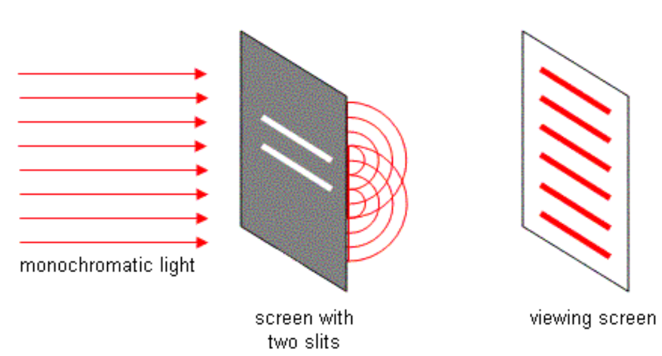Thomas Young’s double-slit diffraction experiment provided clear evidence against Newton’s theory of light. Instead of producing two vertical bright bands right behind the slits, Young observed multiple bright spots spanning a large area than the distance between the slits.

• Diffraction

Despite Newton advocating that light does not exhibit diffraction, experimental evidence from a later time demonstrated that light in fact does diffract.

When light passes through a large or moderately sized opening, it creates an image that can be justified by the corpuscular model as it obeys particles’ rectilinear motion.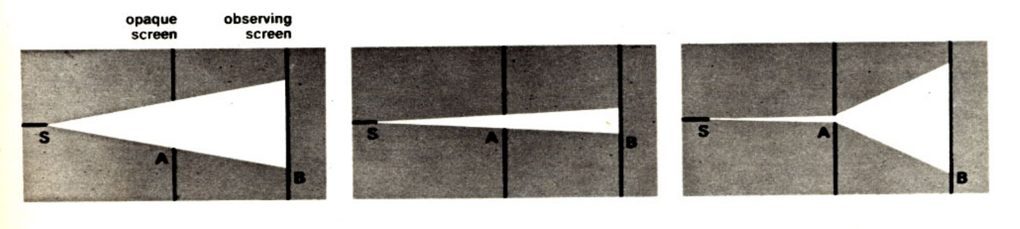However, when a beam of light passes through a small opening or slit, it scatters to form a large image. Newton’s corpuscular model cannot explain this.

• Polarisation

Newton could not elucidate polarisation as a phenomenon (more on this in the next section)

### Huygens’ Wave Model of Light

Huygens' model of light assumes light behaves as a longitudinal wave.

Huygens understood light’s propagation as a wave’s direction of travel. He proposed that the nature of light can be better understood by observing the motion of water surfaces.

• Oscillation
• Crests
• Troughs
• AmplitudeIn addition, he extended the understanding of light as a wave by proposing that a primary wave front can be perceived as infinite number of points, each point generating a secondary wavelet in a spherical manner. The interference of these secondary wavelets create wavefronts of varying shapes and direction.

#### Advantages of Huygens’ Wave Model

At the time of this proposal, Huygens was able to explain most of the properties of light including refraction, reflection & partial refraction and reflection, as well as diffraction.

• Huygens provided a new understanding of optics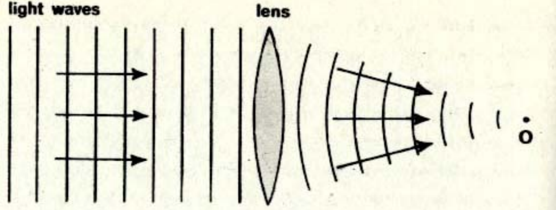Light as a wave travels slower in heavier media such as glass. Huygens explained that this is why light’s path changes as it moves through lenses. As such, light waves which spend longer time in a denser medium would be impeded to a greater extent.

• Reflection is more validly understood compared to Newton’s theory

Reflection of light in the wave model can be understood as wavelets partially propagating back into the original medium.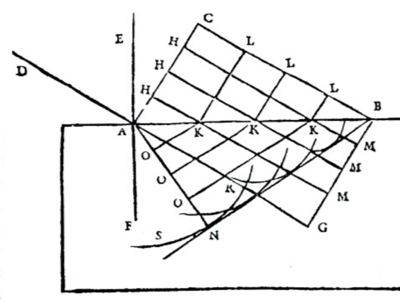• Refraction

By the same principle of reflection, wavelets can also partially propagate into the new medium. Depending on the nature of the new medium, wavelets may travel at a different speed, and thus at a different angle to the normal. This idea explains the phenomenon of refraction.

Huygens’ explanation of reflection and refraction gave rise to a clear understanding of partial refraction and reflection. Depending on the position of secondary wavelets, they may be reflected or continue to propagate into the medium, undergoing refraction.

• Huygens’ wave model supported observations of light’s diffractionHuygens’ principle (wavefront is composed of infinite number of circularly propagating wavelets) combined with Fresnel’s principle of interference provided a clear explanation of light’s diffraction pattern when it passes through a single or multiple slit.

• Polarisation (details included in the next syllabus dot point)

The electromagnetic wave model was able to account for polarisation as one of the many characteristics of light. However, Huygens' longitudinal wave model is unable to explain polarisation as longitudinal waves cannot be polarised.

#### Limitations of the Wave Model of Light (Maxwell & Huygens)

The wave model could not explain quantum mechanical phenomena of light including the photoelectric effect, Compton effect and Raman effect

• Photoelectric effect

A major breakdown of the wave theory occurred in the late 1880s when scientists first discovered that, under certain conditions, light could eject electrons from the atoms of several metals.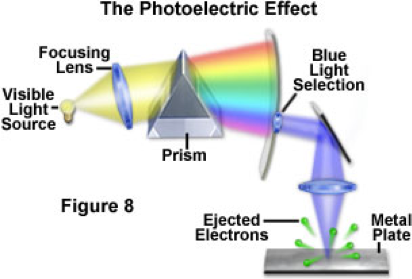German physicist Philipp Lenard became interested in these observations, which he termed the photoelectric effect. Lenard used a prism to split white light into its component colours, and then selectively focused each color onto a metal plate to expel electrons.

For a specific wavelength of light (blue, for example), the electrons produced a constant potential, or a fixed amount of energy. Decreasing or increasing the amount of light produced a corresponding increase or decrease in the number of electrons liberated, but each still maintained the same energy.

In other words, emitted electrons' kinetic energy is dependent on the wavelength of light rather than its intensity. This idea challenged the wave model as supporters believed that energy of light was solely dependent on its intensity.

### Foucault's Experiment

#### Determination of speed of light contributed to the shift from Newton's corpuscular model of light to Huygens' wave model.

Foucault measured the speed of light in an experiment involving mirrors.

Newton proposed the boundary between adjacent media would exert a force on light corpuscles therefore causing them to accelerate. When light corpuscles enter a medium with greater refractive index (e.g. from air to water), the force of attraction is stronger and thus they will increase in velocity.

In contrast, Huygens proposed that light waves would travel slower in a medium with greater refractive index. This was experimentally validated Foucault as he determined that the speed of light in water is slower than in air.

Previous section: Diffraction of Light

Next section: Polarisation of Light